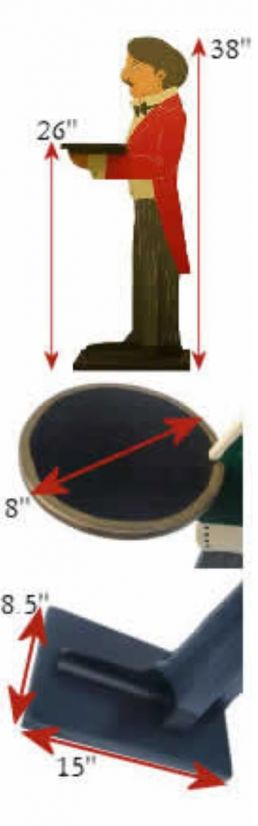Butler Specifications

Individual Butler dimensions can be found on each Models page.Below will give you the standard dimensions for all models.

• Apart from the Guardsman, all the models are 38" tall and 15" wide.
• All soldiers are 48"tall.
• Their trays are 8" in diameter, large enough for a plate or ice bucket.
• Their Plinths are 8.5" X 15" and are made of pine.
• The height of the tray is 26" for all models apart from Luigi's tray which is 30", and the soldiers' which are 32".
• The average weight of each Butler is 3.44Kg.
• The maximum weight the tray can hold is 10Kg.Sizes & Dimensions

Individual Butler dimensions can be found on each Models page.Below will give you the standard dimensions for all models.

Sizes & Dimensions

Individual Butler dimensions can be found on each Models page.Below will give you the standard dimensions for all models.

• Apart from the Guardsman, all the models are 38" tall and 15" wide.
• All soldiers are 48"tall.
• Their trays are 8" in diameter, large enough for a plate or ice bucket.
• Their Plinths are 8.5" X 15" and are made of pine.
• The height of the tray is 26" for all models apart from Luigi's tray which is 30", and the soldiers' which are 32".
• The average weight of each Butler is 3.44Kg.
• The maximum weight the tray can hold is 10Kg.Happy Owners